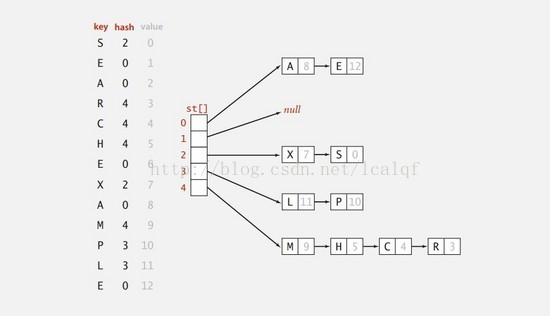（本文转载，自己做了少许修正，若有侵权请联系删除）

## 简单了解散列（hash）算法思想#

``````//下面是实现的代码
int m,n,hashTable = {0};
cin >>m>>n; //m和n指的是集合M和集合N的元素个数
for (int i = 0,x;i<m ;i++ ){
cin>>x;
hashTable[x] = 1;
}
for (int i = 0,x;i<n ;i++ ){
cin>>x;
if(hashTable[x] == 1){
cout<<"YES";
}else{
cout<<"NO";
}
}
``````

## 先是整数hash#

### 除留取余法#

``````H(key) = key%mod;//mod 是选定的整数
``````

### 拉链法### 字符串hash(太妙了)#

``````int hashFunc(char c[] ,int len){
int id = 0;
for (int i = 0;i<len ;i++ ){
id = id*26 + (c[i] - 'A');
}

return id;
}
``````

``````int hashFunc(char c[] ,int len){
int id = 0;
for (int i = 0;i<len ;i++ ){
if(c[i]>='A'&&c[i]<='Z'){
id = id*52 + (c[i] - 'A');
}else if(c[i]>='a'&&c[i]<='z'){
id = id*52 + (c[i] - 'a') + 26;
}

}

return id;
}
``````

1. 按照上述方法，增加进制到62；
2. 如果末位确定是个数字，那么前面的字母转化成整数后直接拼接上这个数字，例如BCD4，BCD转化为731，拼接上后面的4就是7314。
``````int hashFunc(char c[] ,int len){
int id = 0;
for (int i = 0;i<len ;i++ ){
id = id * 26 + (c[i] - 'A');
}
id = id * 10 + (c[len - 1] - '0');

return id;
}
``````

hash表拉链法解决冲突